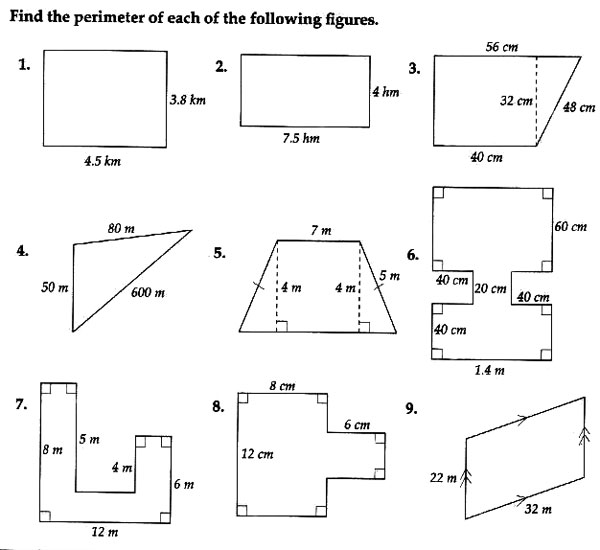Assessment Questions

Questions 1 - 14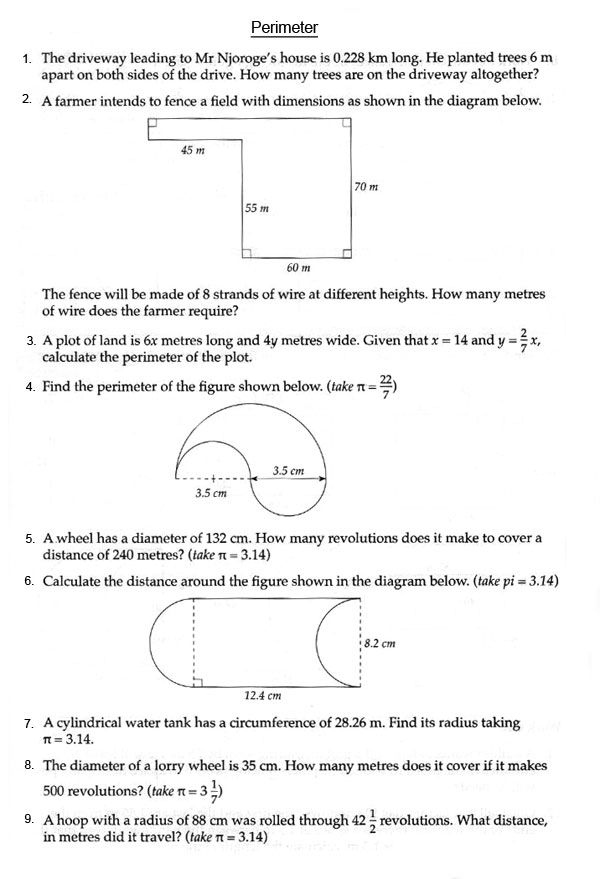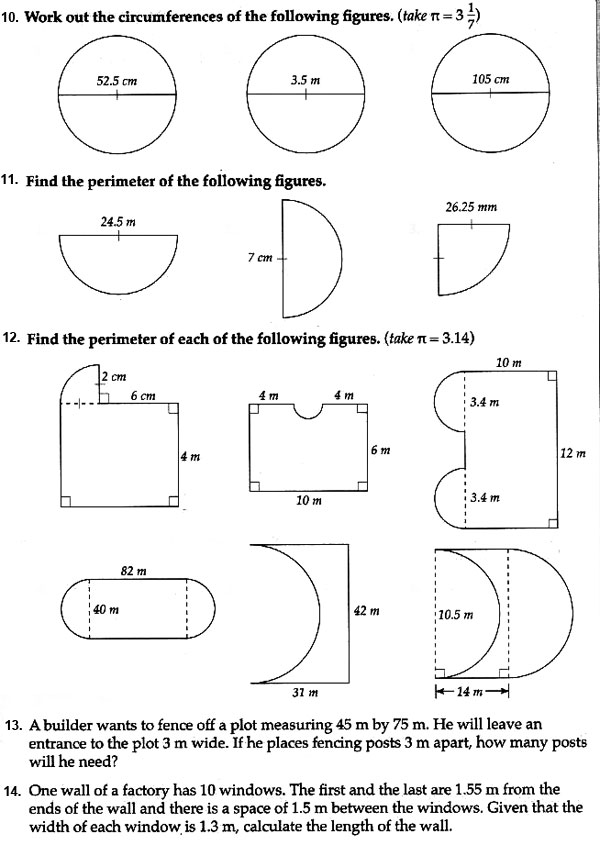15. Find the perimeter of the shape given below. Give the answer in meters. Take $$\pi = {22\over7}$$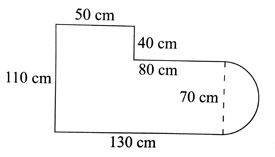16. An ostrich walks on a path that is 3.6 km long. It makes steps of 1m 20 cm. How many steps does it have to make to cover the path?

17. A wheel makes 500 revolutions in order to cover a distance of 1km 884m. Calculate the diameter of the wheel in meters and centimetres. ($$Take \quad \pi = {22\over7}$$)

18. The perimeter of a square is 56.12 m, find the length of one of its sides.

19. The figure below shows a rectangular farm.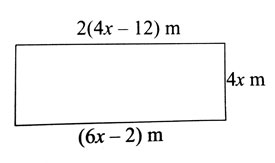Fin its perimeter.

20. Work out;

 m cm 3 90 X 3

21. Onyango had 5 pieces of materials each measuring 7 km 750 m. If he joined them together, what was his new length?

22. Find the perimeter of the figure  given below.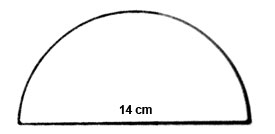Question 23